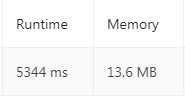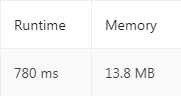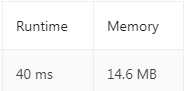specific ext i+1 bre leetcode amp two sum {} span

refer to https://blog.csdn.net/linfeng886/article/details/79772348

Description

Given an array of integers, return indices of the two numbers such that they add up to a specific target.

You may assume that each input would have exactly one solution, and you may not use the same element twice.

Example:

Given nums = [2, 7, 11, 15], target = 9, Because nums + nums = 2 + 7 = 9，return [0, 1].

Python code

1. Brute Force

```class Solution:
def twoSum(self, nums: List[int], target: int) -> List[int]:
N = len(nums)
for i in range(N):
for j in range(i+1, N): # Traverse the next element in turn
if nums[j] == target - nums[i]:
return [i,j]
break
else:
continue```This methods have high computation complexity and memory storage.

2. one for loop

```class Solution:
def twoSum(self, nums: List[int], target: int) -> List[int]:
N = len(nums)
for i in range(N):
rest = target - nums[i]
if rest in nums:
j = nums.index(rest)
return [i,j]
break
else:
continue```3. create a dict first

```class Solution:
def twoSum(self, nums: List[int], target: int) -> List[int]:
N = len(nums)
d = {}
for i in range(N):
rest = target - nums[i]
if nums[i] in d:
return d[nums[i]], i
else:
d[rest] = i```Leetcode 1. Two Sum (Python)